# Extreme gaps between consecutive zeros of the zeta-function

The number of zeros of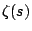with imaginary parts smaller than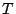is given by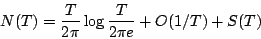where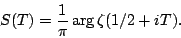It is known that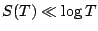and, conditional on the Riemann Hypothesis that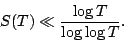Thus, the average gap between zeros ofat heightis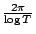and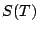measures the local fluctuations in the zero spacings. (If not forthe zeros ofwould have a picket fence' spacing.)

Clearly, a large gap between consecutive zeros ofimplies thatis correspondingly large. It seems not unreasonable to speculate that the largest gaps between consecutive zeros ofwill match' with the largest values of values of: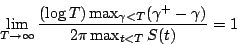Thus, if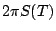is occasionally as large as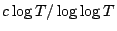see the article on $S(T)$ then we would expect the maximal gaps between zeros of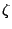to be as large as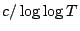.

Back to the main index for L-functions and Random Matrix Theory.